# Class 9 RD Sharma Solutions – Chapter 16 Circles- Exercise 16.4

• Last Updated : 04 May, 2021

### Question 1. In Fig., O is the centre of the circle. If ∠APB∠APB= 50°, find ∠AOB and ∠OAB.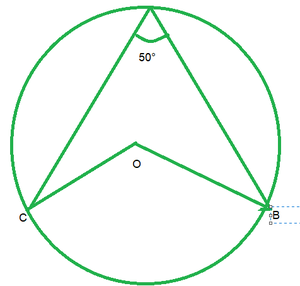Solution:

∠APB=50°

By degree measure theorem

∠AOB=2APB

∠APB=2*50°=100°

Then ∠OAB=∠OBA            [Angles opposite to equal sides]

Let ∠OAB=x

In △OAb, by angle sum property

∠OAB+∠OBA+∠AOB=180°

x+x+100°=180°

2x=180°-100°

2x=80°

x=40°

∠OAB=∠OBA=40°

### Question 2. In Fig., it is given that O is the centre of the circle and ∠AOC = 150°. Find ∠ABC.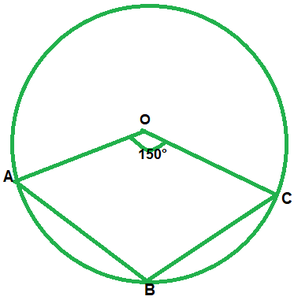Solution:

∠AOC = 150°

∠AOC +reflex ∠AOC = 360°             [complex angle]

150°+reflex ∠AOC = 360°

reflex ∠AOC=210°

2∠ABC=210°          [By degree measure theorem]

∠ABC=210°/2=105°

### Question 3. In Fig., O is the centre of the circle. Find ∠BAC.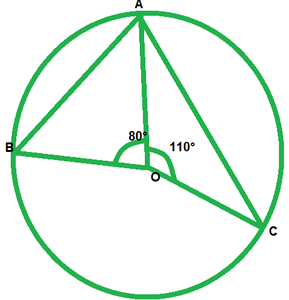Solution:

We have ∠AOB=80°

And ∠AOC=110°

Therefore, ∠AOB+∠AOC+∠BOC=360°          [complete angle]

80+100+∠BOC=360°

∠BOC=360°-80°-110°

∠BOC=70°

By degree measure theorem

∠BOC=2∠BAC

170=2∠BAC

∠BAC=170°/2=85°

### Question 4. If O is the centre of the circle, find the value of x in each of the following figures.

Solution:

i)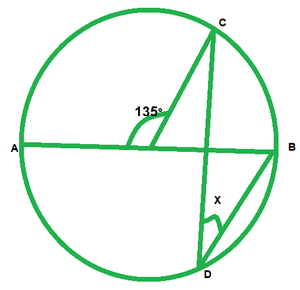∠AOC=135°

∠AOC+BOC=185°             [Linear pair of angles]

135°+∠BOC=180°

∠BOC=180°-135°=45°

By degree measure theorem

∠BOC=2∠COB

45=2x

x=45°/2=22\frac{1}{2}

ii)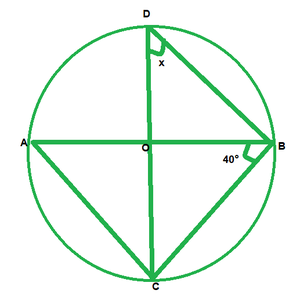We have

∠ABC=40°

∠ACB=90°               [Angle in semicircle]

In △ABC, by angle sum property

∠CAB+∠ACB+∠ABC=180°

∠CAB+90°+40°=180°

∠CAB=180°-90°-40°

∠CAB=50°

Now,

∠CDB=∠CAB         [Angle is same in segment]

x=50°

iii)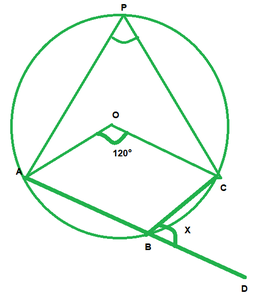We have,

∠AOC=120°

By degree measure theorem

∠AOC=2∠APC

120°=2∠APC

∠APC=120°/2=60

∠APC+∠ABC=180°      [Opposite angles of cyclic quadrilaterals]

60°+∠ABC=180°

∠ABC=180°-60°

∠ABC=120°

∠ABC+∠DBC=180°      [Linear pair of angles]

120°+x=180°

x=180°-120°=60°

iv)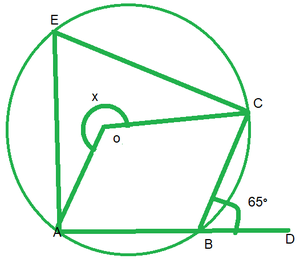We have

∠CBD=65°

∠ABC+∠CBD=180°      [Linear pair of angles]

∠ABC=65°=180°

∠ABC=180°-65°=115°

reflex ∠AOC=2∠ABC          [By degree measure theorem]

x=2*115°

x=230°

v)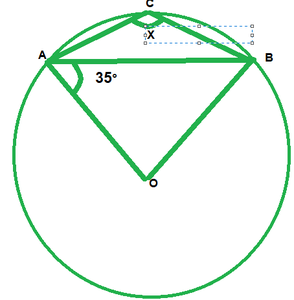We have,

∠OAB=35°

Then, ∠OBA=∠OAB=35°      [Angles opposite to equal radii]

In △AOB, by angle sum property

∠AOB+∠OAB+∠OBA=180°

∠AOB+35°+35°=180°

∠AOB=180°-35°=110°

∠AOB+reflex ∠AOB=360°             [complex angle]

110+reflex∠AOB=360°

reflex∠AOB=360°-110°=250°

By degree measure theorem reflex∠AOB=2∠ACB

250°=2x

x=250°/2=125°

vi)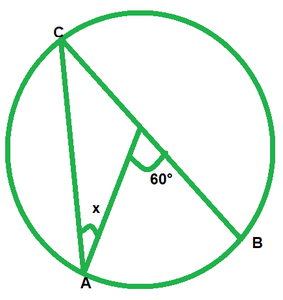We have,

∠AOB=60

By degree measure theorem reflex

∠AOB=2∠ACB

60=2∠ACB

∠ACB=60°/2=30°         [Angle opposite to equal radii]

x=30°

vii)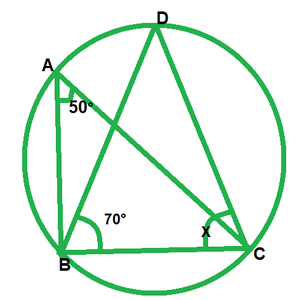We have,

∠BAC=50°  and  ∠DBC=70°

∠BDC=∠BAC=50°           [Angle in same segment]

In △BDC, by angle sum property

∠BDC+∠BCD+∠DBC=180°

50°+x+70°=180°

x=180°-50°-70°=60°

viii)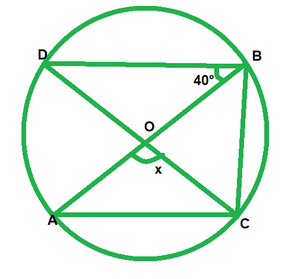We have,

∠DBO=40° and ∠DBC=90°        ——-[Angle in a semi circle]

∠DBO+∠OBC=90°

40°+∠OBC=90°

∠OBC=90°-40°=50°

By degree measure theorem

∠AOC=∠OBC

x=2*50°=100°

ix)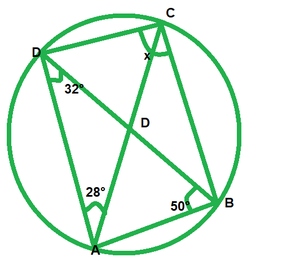In ∆DAB, by angle sum property

32°+∠DAB+50°=180°

∠DAB=180°-32°-50°

∠DAB=98°

Now,

∠OAB+∠DCB=180°          [opposite angle of cyclic quadrilateral]

98°+x=180°

x=180°-98°=82°

x)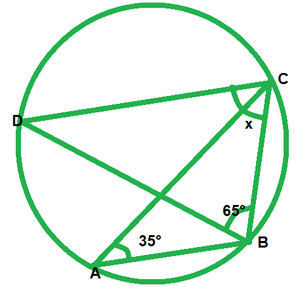We have,

∠BAC=35°

∠BDC=∠BAC=35°            [Angle in same segment]

In ∆BCD, by angle sum property

∠BDC+∠BCD+∠DBC=180°

35°+x+65°=180°

x=180°-35°-65°=80°

xi)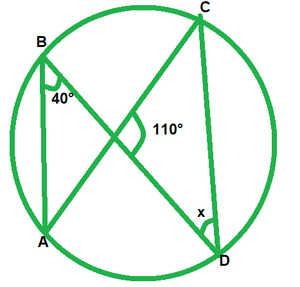We have,

∠ABD=40°

∠ACD=∠ABD=40°         [Angle in same segment]

In ∆PCD, by angle sum property

∠PCD+∠CPO+∠PDC=180°

40°+110°+x=180°

x=180°-150°

x=30°

xii)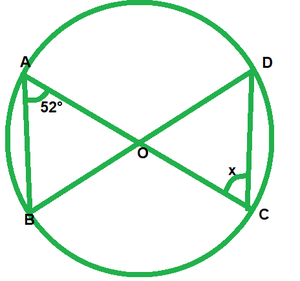Given that,

∠BAC=52°

Then ∠BDC=∠BAC=52°        [Angle in same segment]

Since OD=OC

Then ∠ODC=∠OCD            [ Opposite angle to equal radii]

x=52°

### Question 5. O is the circumcenter of the triangle ABC and OD is perpendicular on BC. Prove that ∠BOD = ∠A.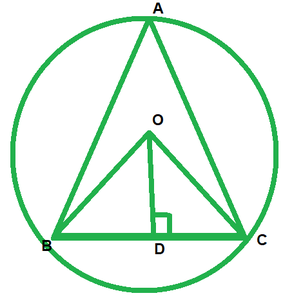Solution:

We have to prove that  ∠BOD=∠A

since, circum center  is the intersection of perpendicular bisector of each side of the the triangle. Now according to figure A,B,C are the vertices of ∆ABC

In ∆BOC, OD is perpendicular  bisector  of BC.

so, BD=CD

OB=OC        ——–(Radius of the same circle)

And,

OD=OD            —–[common]

Therefore,

∆BDO≅∆CDO              (SSS concurrency criterion )

∠BOD=∠COD              (by cpct)

We know that angle formed any chord  of the circle at the center is twice of the  angle formed at  the circumference  by same chord

Therefore,

∠BAC=\frac{1}{2}  ∠BOC

∠BAC=\frac{1}{2}*2∠BOD

∠BAC=∠BOD

Therefore,

∠BOD=∠A

### Question 6. In Fig., O is the centre of the circle, BO is the bisector of ∠ABC. Show that AB = AC.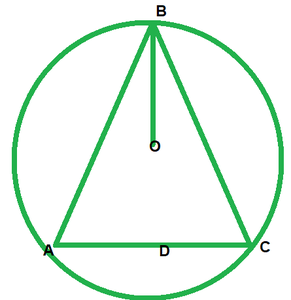Solution:

Given, BO is the bisector of ∠ABC

To prove: AB=BC

Proof:  Since, BO is the bisector  of ∠ABC.

Then, ∠ABO=∠CBO        —-(i)

Then, ∠ABO=∠DAB           ——–(ii)    [opposite angles to equal sides]

Then, ∠OAB=∠OCB       ——–(iii)  [opposite angles to equal sides]

compare equations  (i), (ii) and (iii)

∠OAB=∠OCB ——-(iv)

In ∆OAB  and   ∆OCB

∠OAB=∠OCB       [From(iv)]

∠OBA=∠OBC          [Given]

OB=OB           [common]

Then

∆OAB≅∆OCB        [By AAS condition]

Therefore, AB=BC      [CPCT]

### Question 7. In Fig., O is the centre of the circle, prove that ∠x = ∠y + ∠z.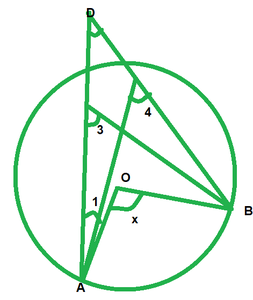Solution:

We have,

∠3=∠4            [Angles in same segment]

∠x=2∠3          [By degree measure theorem]

∠x=∠3+∠3⇒∠x=∠3+∠4 ——–(i)        [∠3=angle 4]

But ∠y=∠3+∠1          [By exterior angle property]

⇒∠3=∠y-∠1    —-(ii)

from (i) and (ii)

∠x=∠y-∠1+∠4

∠x=∠y+∠4-∠1

∠x=∠y+∠z+∠1-∠1           [By exterior angle property]

∠x=∠y+∠z

### Question 8. In Fig., O and O’ are centres of two circles intersecting at B and C. ACD is a straight line, find x.Solution:

By degree measure theorem

∠AOB=2∠ACB

130°=2∠ACB⇒∠ACB=130°/2=65

∠ACB+∠BCD=180°        [Linear pair of angles]

65°+∠BCD=180°

∠BCD=180-65=115

By degree measure theorem

reflex∠BOD=2∠BCD

reflex∠BOD=2*115°=230°

Now, reflex∠BOD+∠BOD=360°       [complex angle]

230°+x=360°

x=360°-230°

x=130°

### Question 9. In Fig., O is the centre of a circle and PQ is a diameter. If ∠ROS = 40°, find ∠RTS.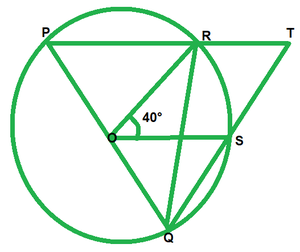Solution:

Since PQ is diameter

Then,

∠PRQ=90°          [Angle in semi circle]

∠PRQ+∠TRQ=180°           [Linear pair of angle]

90+∠TRQ=180

∠TRQ=180°-90°=90°

By degree measure  theorem

∠ROS=2∠RQS

40=2∠RQS

∠RQS=40°/2=20°

In ∆RQT, by angle  sum property

∠RQT+∠QRT+∠RTS=180°

20°+90°+∠RTS=180°

### Question 10. In Fig., if ∠ACB = 40°, ∠DPB = 120°, find ∠CBD.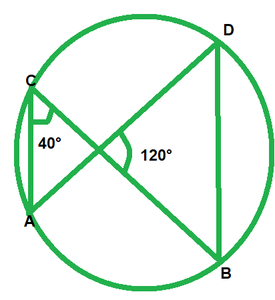Solution:

We have,

∠ACB=40°;  ∠DPB=120°

∠APB=∠DCB=40°            [Angle in same segment]

In ∆POB, by angle sum property

∠PDB+∠PBD+∠BPD=180°

40+∠PBD+120°=180°

∠PBD=180°-40°-120°

∠PBD=20°

∠CBD=20°

### Question 11. A chord of a circle is equal to the radius of the circle. Find the angle subtended by the chord at a point on the minor arc and also at a point on the major arc.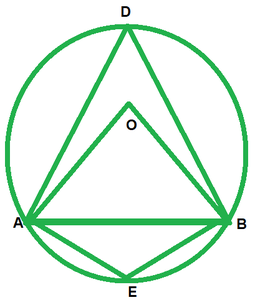Solution:

Construction: O is center and r is radius and given that chord is equal to radius of circle.

Now in ∆AOB we have

AO=OB=BA         (It is given that chord is equal to radius of circle)

so, ∆AOB is an equilateral triangle

∠AOB=60°

So, ∠AOB=2∠ADB     (The angle subtended by an arc of a circle at the center  is double the angle subtended by it at any point  on the remaining  prat of the circle)